# RD Sharma Class 12 and Chapter Indefinite Integrals Maths Solutions

## Maths Class 12 and Chapter Indefinite Integrals RD Sharma SolutionsFilter
Filters :
CLASSES
•
•
•
•
•
•
•
CHAPTERS
•
• 24 More

Students are mostly asked to study from their course books and not invest time in finding different books as it will only waste their time. But, that's not what experts believe and pass on nowadays.

Some of the experts who are quite experienced in the educational field say that it is more important for students to have a clear base and hands-on practice, which altogether prepare them not only for CBSE board exams but also to crack any competitive exams.

Being a student, you must feel complexity in solving some questions as the formulas provided in NCERT books are limited. But, when it comes to maths, the syllabus and concepts are wide. Only a book with continuous updating and variety of concepts can help a student to learn beyond.

R D. Sharma is one of the most popular conceptual learning books that allow students to start right from the basics. Most of the students even find it difficult to raise their doubts in class too or tuitions as they feel hesitate of what other fellow mates think of them.

With the help of R.D. Sharma books, students can neglect this feeling of missing out and can revise their base as many times as they want.

Detailed concepts and multiple questions are yet another reason to opt these books as each question focused on a different level of aptitude that prepares you well-in-advance for competitive exams like JEE Mains

INTRODUCTION:
• If the integration is a derivative of a known function, then the equivalent indefinite integral can be directly estimated.
• If the integration is not a derivative of a known function, the integral may be estimated with the help of any of the following three rules:

1) Integration by substitution or by modification of the independent variable.

2) Integration by parts
3) Integration by partial fractions

### INDEFINITE INTEGRALS WHICH CAN BE ESTIMATED BY DIRECT SUBSTITUTIONS:

1) If integral is in the form ∫ f(g(x)) g'(x) dx, then place g(x) = t, provided ∫ f(t) exists.

2) ∫ f'(x)/f(x) dx = ln |f (x)| + c, By, substituting f (x) = t => f' (x) dx = dt

=> ∫ dt/t = ln |t| + c = ln |f (x)| + c.

3) ∫ f'(x)√f(x) dx = a pair of √f(x)+c, place f (x) = t

Then ∫ dt/√t = 2√t + c = 2√f(x) + c.

### INDEFINITE INTEGRALS WHICH CAN BE ESTIMATED BY DIRECT SUBSTITUTIONS:

1) If the integral is in the form of ∫ f(g(x)) g'(x) dx, then put g(x) = t, provided ∫ f(t) exists.
2) ∫ f'(x)/f(x) dx = ln |f (x)| + c, By putting f (x) = t => f' (x) dx = dt
=> ∫ dt/t = ln |t| + c = ln |f (x)| + c.
3) ∫ f'(x)√f(x) dx = 2 √f(x)+c, Put f (x) = t
Then ∫ dt/√t = 2√t + c = 2√f(x) + c.

### SOME STANDARD SUBSTITUTIONS:

1) If the term is in form x2 + a2 or √x2 + a2, place x = a tanθ or a cotθ

2) If the term is in form x2 - a2 or √x2 – a2, put x = a sec θ or a cosecθ

3) If the term is in form a2 - x2 or √x2 + a2, place x = a sin θ or a cosθ

4) If each √a+x, √a–x, are present, then put x = a cos θ.

5) If the term is in form √(x–a)(b–x), put x = a cos2θ + b sin2θ
6) For the type (√x2+a2±x)n or (x±√x2–a2)n, put the expression within the bracket = t.
7) For 1/(x+a)n1 (x+b)n2, where n1,n2 N (and > 1), again put (x + a) = t (x + b)

• If the integrand is of the shape f(x)g(x), where g(x) is a function of the integral of f(x), then put integral of f(x) = t.

• The integral of a product of 2 functions of x is evaluated with the assistance of integration by elements.
Let u and v be 2 functions of x, then ∫uv dx = u∫v dx - ∫[du/dx ∫v dx]dx

• If there's no second perform out there, then unity is taken as the second function.
• In the integral ∫g(x)exdx, if g(x) can be expressed as g(x) = f(x) + f'(x) then ∫g(x)ex dx=∫ ex [f(x) + f'(x)] dx = exf(x) + c

• to jot down P(x)/ Q(x) in partial fractions, write Q(x) in the form Q(x) = (x – a)k ... (x2 + αx + β)r ... where binomials are different, and

then set
Where A1, A2, ...Ak, M1, M2, ......, Mr, N1, N2, .... ,Nr square measure real constants to be determined.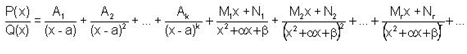• A rational perform P(x)/Q(x) is correct if the degree of polynomial Q(x) is larger than the degree of the polynomial P(x).
• If the degree of P(x) is greater than or equal to the degree of Q(x), we first write P(x)/Q(x) = h(x) + P(x)/Q(x), where h(x) is a polynomial and p(x) is a polynomial of degree less than the degree of polynomial Q(x).

### IN INTEGRALS OF THE FORM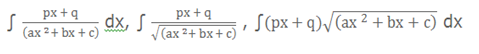1) put, px + q = l

2) To find l and m we must compare the coefficient of x and constant term on both sides of the identity.

3) then the problem will reduce to the sum of two integrals which can be integrated easily.

### IN CASE OF INTEGRALS OF THE TYPE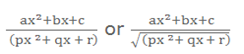1) Substitute ax2 + bx + c = M (px2 + qx + r) + N (2px + q) + R.
2) Find M, N, & R.

3) the combination reduces to the integration of 3 independent functions.

• The Irrational functions in the form (ax+b)1/n and x can be easily evaluated by the substitution = ax+ b. Thus ∫f(x, (ax + b)1/n)dx = ∫ f ((tn – b)/ a , t) ntn-1 / a dt
• In case of integrals of the form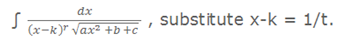### TO CALCULATE THE INDEFINITE INTEGRALS OF THE FORM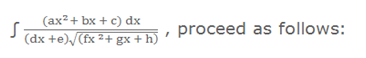1) Write, ax2 +bx+c = A1 (dx +e) (2fx +g) +B1(dx +e) +C1where A1, B1 and C1 are constants which can be obtained by comparing the coefficient of like terms on both sides.

2) The given integral can reduce its form then,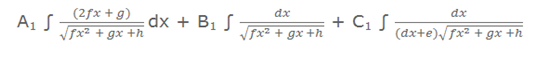1) If R(-sin x, cos x) = -R(sin x, cos x), substitute cos x = t
2) If R(sin x, -cos x) = -R(sin x, cos x), substitute sin x = t
3) If R(-sin x, - cos x) = R(sin x, cos x), substitute tan x = t

### THE IMPORTANT INDEFINITE INTEGRAL FORMULAE ARE:

1) ∫ exdx = ex + c

2) ∫ 1/x dx = ln |x| + c

3) ∫ ax dx = ax/ ln a + c (a > 0)

4) ∫ cos x dx = sin x + c

5) ∫ sin x dx = - cos x + c

6) ∫ sec2 x dx = tan x + c

7) ∫ cosec x cot x dx = - cosec x + c

8) ∫sec x tan x dx = sec x + c

9) ∫ cosec2 x dx = - cot x + c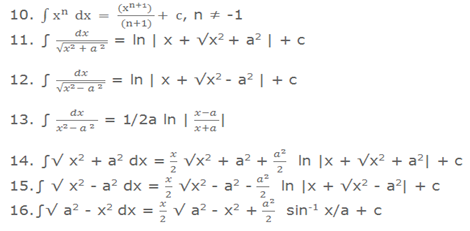### INTEGRATION BY PARTS:

This is a method used to integrate the product of two functions. If f(x) and g(x) will be integrated then,

f(x) g(x) dx = f(x) ∫ g(x) dx – ∫ {d/dx (f(x)) . ∫g(x) dx} dx.

• For selecting the first function, the following order is followed:

Inverse → Logarithmic → Algebraic → Trigonometric → Exponential

### INTEGRATION USING PARTIAL FRACTIONS:

• If f(x) and g(x) ar 2 polynomials, and deg (f(x)) < deg (g(x)), then f(x)/g(x) is called a proper rational fraction.

• If deg (f(x)) ≥ deg (g(x)), then f(x)/g(x) is called an improper rational fraction.
If f(x)/g(x) is an improper rational function, then divide f(x) by g(x) and convert it to proper rational function i.e. f(x) / g(x) = l(x) + h(x)/g(x).
Any proper rational function f(x)/g(x) can be expressed as the sum of rational functions each having a factor of g(x).

• Each of those factors is named a partial fraction and therefore the method of getting them is named decomposition of f(x)/g(x) into partial fractions.

## SIGNIFICANCE OF RD SHARMA CLASS 12TH MATH

1. The twelfth standard Mathematics RD Sharma gives understudies right ideas from the base and helps to comprehend the points in a wide way.

2.The competitors who always alluded RD Sharma help guides for discharge the course to advance since it gives basic thoughts as opposed to sounding the formula-based request clearly.

3. We all understand that maths isn't composing type subject that we can learn Instead, it is a subject that requires fortunate learning of plans and practicing the request as much as an applicant gauge grant.

4.RD Sharma twelfth class maths books have different practice questions and exercises to gauge to more promptly comprehend the essential thought of request and how to settle it.

5. Abstracted and accumulated via preparing section experts, these books are sorted out as per the present test plans. The new arrival of the book contains all the continuous changes and are twofold checked by experienced teachers.

6. As the book includes both, appreciated and unsolved request, the hopefuls can find the plans of such practice rehearses online too. In this way, RD Sharma books are amassed agreeably and bolstered with online courses of action.